# Maharashtra Board Class 6 Maths Solutions Chapter 1 Basic Concepts in Geometry Practice Set 1

## Maharashtra State Board Class 6 Maths Solutions Chapter 1 Basic Concepts in Geometry Practice Set 1

Question 1.
Look at the figure alongside and name the following:
i. Collinear points
ii. Rays
iii. Line segments
iv. Lines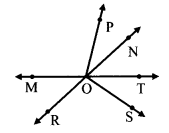Solution:

 i. Collinear Points Points M, 0 and T Points R, O and N ii. Rays ray OP, ray OM, ray OR, ray OS, ray OT and ray ON iii. Line Segments seg MT, seg RN, seg OP, seg OM, seg OR, seg OS, seg OT and seg ON iv. Lines line MT and line RN

Question 2.
Write the different names of the line.Solution:
The different names of the given line are line l, line AB, line AC, line AD, line BC, line BD and line CD.

Question 3.Solution:
(i – Line),
(ii – Line Segment),
(iii – Plane),
(iv – Ray)

Question 4.
Observe the given figure. Name the parallel lines, the concurrent lines and the points of concurrence in the figure.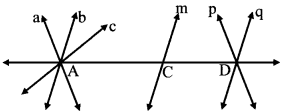Solution:

 Parallel Lines line b, line m and line q are parallel to each other. line a and line p are parallel to each other. Concurrent Lines and Point of Concurrence line AD, line a, line b and line c are concurrent. Point A is their point of concurrence. line AD, line p and line q are concurrent. Point D is their point of concurrence.

Maharashtra Board Class 6 Maths Chapter 1 Basic Concepts in Geometry Intext Questions and Activities

Question 1.
Complete the rangoli. Then, have a class discussion with the help of the following questions:

1. What kind of surface do you need for making a rangoli?
2. How do you start making a rangoli?
3. What did you do in order to complete the rangoli?
4. Name the different shapes you see in the rangoli.
5. Would it be possible to make a rangoli on a scooter or on an elephant’s back?
6. When making a rangoli on paper, what do you use to make the dots?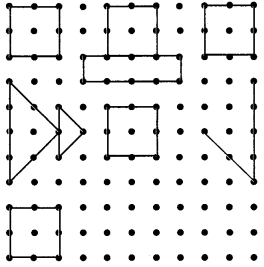Solution:

1. For making a rangoli, I need a flat surface.
2. I can start making a rangoli by drawing equally spaced dots on the flat surface using a chalk.
3. In order to complete the rangoli, I joined the dots by straight lines to make a design.
4. In the rangoli, I find various shapes such as square, rectangle and triangles of two different size.
5. No. It won’t be possible to make a rangoli on a scooter or on an elephant’s back as they do not have a flat surface.
6. When making a rangoli on paper, I made use of scale and pencil to make equally spaced dots.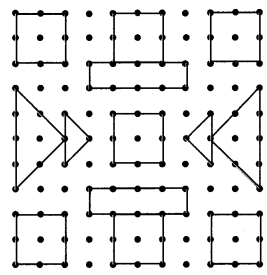Question 2.
Write the proper term, ‘intersecting lines’ or ‘parallel lines’ in each of the empty boxes. (Textbook pg. no. 4)Solution:
i. Intersecting Lines
ii. Parallel Lines
iii. Intersecting Lines

Question 3.
Draw a point on the blackboard. Every student now draws a line that passes through that point. How many such lines can be drawn? (Textbook pg. no. 2)
Solution:
An infinite number of lines can be drawn through one point.Question 4.
Draw a point on a paper and use your ruler to draw lines that pass through it. How many such lines can you draw? (Textbook pg. no. 2)
Solution:
An infinite number of lines can be drawn through one point.

Question 5.
There are 9 points in the figure. Name them. (Textbook pg. no. 3)
i. If you choose any two points, how many lines can pass through the pair?
ii. Which three or more of these nine points lie on a straight line?
iii. Of these nine points, name any three or more points which do not lie on the same line.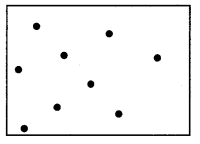Solution:
i. One and only one line can be drawn through two distinct , points.
ii. Points A, B, C and D lie on the same line. Points F, G and C lie on the same line.
iii. Points E, F, G, H and I do not lie on the same line, points A, B, E, H and I do not lie on the same line.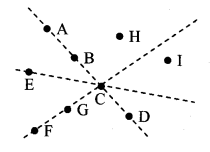Question 6.
Observe the picture of the game being played. Identify the collinear players, non-collinear players, parallel lines and the plane. (Textbook pg. no. 4)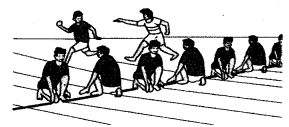Solution:

 i. Collinear Players Players A, B, C, D, E, F, G ii. Non-collinear Players Players I, H, C Players I, A, B etc. iii. Parallel Lines line l, line m, line n, line p, line q, line r and line s iv. Plane The ground on which the boys are playing is the planeQuestion 7.
In January, we can see the constellation of Orion in the eastern sky after seven in the evening. Then it moves up slowly in the sky. Can you see the three collinear stars in this constellation? Do you also see a bright star on the same line some distance away? (Textbook pg. no. 4)Solution:

1. The three stars shown by points C, D and E are collinear.
2. The star shown by point H lies on the same line as the stars C, D and E.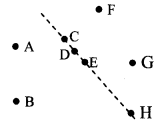Question 8.
Maths is fun! (Textbook pg. no. 5)
Take a flat piece of thermocol or cardboard, a needle and thread. Tie a big knot or button or bead at one end of the thread. Thread the needle with the other end. Pass the needle up through any convenient point P. Pull the thread up, leaving the knot or the button below. Remove the needle and put it aside. Now hold the free end of the thread and gently pull it straight. Which figure do you see? Now, holding the thread straight, turn it in different directions. See how a countless number of lines can pass through a single point P.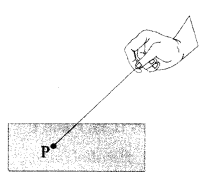Solution:

1. The pulled thread forms a straight line.
2. An infinite number of lines can be drawn through one point.

Question 9.
Choose the correct option for each of the following questions:
i. ______ is used to name a point.
(A) Capital letter
(B) Small letter
(C) Number
(D) Roman numeral
Solution :
(A) Capital letter

ii. A line segment has two points showing its limits. They are called_____
(A) origin
(B) end points
(D) infinite points
Solution :
(B) end points

iii. An arrow head is drawn at one end of the ray to show that it is _____ on that side.
(A) finite
(B) ending
(C) infinite
(D) broken
Solution :
(C) infinite

iv. Lines which lie in the same plane but do not intersect are said to be ____ to each other.
(A) intersecting
(B) collinear
(C) parallel
(D) non-collinear
Solution :
(C) parallel

Question 10.
Determine the collinear and non-collinear points in the figure alongside: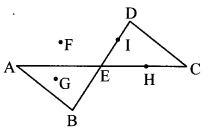Solution:
Collinear points:

1. Points A, E, H and C.
2. Points B, E, I and D.

Non-collinear points:
Points B, G, F and I

Question 11.
Look at the figure alongside and answer the questions given below:
i. Name the parallel lines.
ii. Name the concurrent lines and the point of concurrence.
iii. Write the different names of line PV.Solution:
i. Parallel lines:
a. line l and line n
b. line p, line q, line r and line s

ii. Concurrent Lines: line q, line m, line n
Point of Concurrence: point S

iii. line l, line PT, line PR, line PV, line RT, line RV and line TV.

Question 12.
Name the different line segments and rays in the given figure: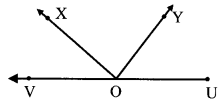Solution:
Line Segments:
seg UV, seg OY, seg OX, seg OV and seg OU

Rays:
ray OV, ray OX, ray OY and ray UV.